# Circle Theorems

GCSELevel 4-5Level 6-7Level 8-9Edexcel iGCSE

## Circle Theorems

Circle theorems are rules used when finding angles and lengths within a circle or parts connected to a circle.

There are $9$ theorems you will need to understand and be able to use.

## Rule 1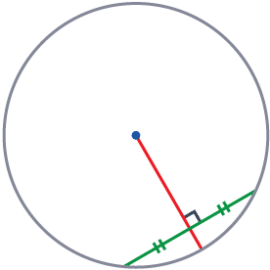Perpendicular Bisector of a Chord

A chord is any line drawn from one point on the circumference to another.

The perpendicular bisector of a chord ALWAYS passes through the centre of the circle.

Level 4-5GCSEEdexcel iGCSE

## Rule 2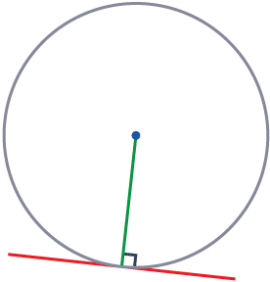Angle between a Radius and Tangent

A tangent to a circle will always meet a radius – as the radius can go from the centre of the circle to anywhere on the circumference.

A tangent and a radius will ALWAYS meet at $90\degree$

Level 4-5GCSEEdexcel iGCSE

## Rule 3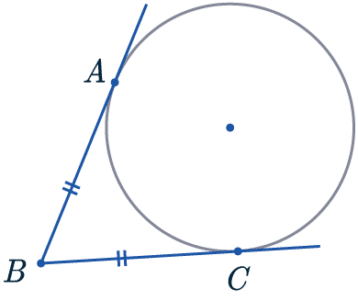Tangents from the Same Point

As there can be multiple tangents to the same point, two tangents can come from the same point and meet the circle at $2$ separate points.

Tangents from the same point to the circle are the same length.

Level 4-5GCSEEdexcel iGCSE

## Rule 4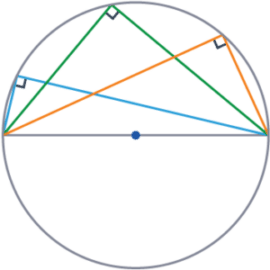Angles in a Semi-Circle

When an angle extends from a diameter, this can be described as the diameter subtends an angle at the circumference.

The angle subtended from the diameter within a semi-circle is $90\degree$

Level 6-7GCSEEdexcel iGCSE

## Rule 5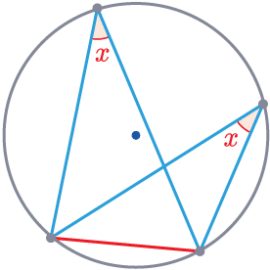Angle in the Same Segment

When a chord is drawn in a circle, both sides of the chord are segments.

When triangles are drawn from the chord in the same segment, the angles at the circumference are equal.

Level 6-7GCSEEdexcel iGCSE

## Rule 6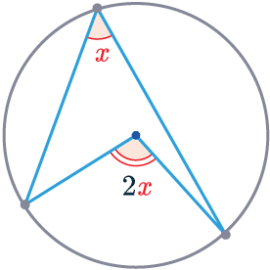Angle at the Centre

This rule occurs when an angle at the centre and an angle at the circumference are subtended from the same two points on the circumference.

The angle at the centre of the circle is double the size of the angle at the circumference.

Level 6-7GCSEEdexcel iGCSE

## Rule 7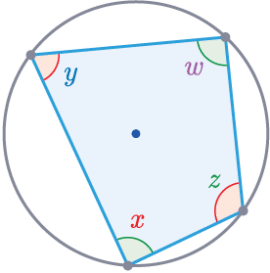A cyclic quadrilateral is a four-sided shape, where each vertex touches the circumference of a circle.

In a cyclic quadrilateral, opposite angles ALWAYS add to $180\degree$

$\textcolor{purple}{w}+\textcolor{red}{x}=180\degree$

$\textcolor{blue}{y}+\textcolor{limegreen}{z}=180\degree$

Level 6-7GCSEEdexcel iGCSE

## Rule 8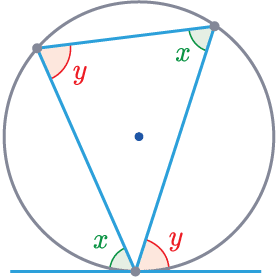Alternate Segment Theory

This rule is relevant where there is a triangle within a circle with a tangent to the circle at one of the points.

The angle between the tangent and the side of the triangle is equal to the opposite angle of the triangle.

Level 6-7GCSEEdexcel iGCSE

## Rule 9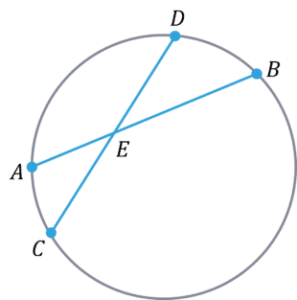Intersecting chords

This rule is made of $2$ properties surrounding chords.

1. Internal Intersection

For any two chords that insect within a circle:

$AE\times EB=CE\times ED$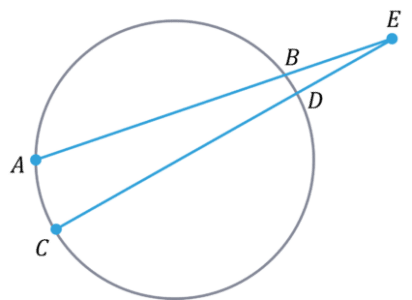2. External Intersection

For any two chords are extended and meet outside a circle:

$AE\times BE=CE\times DE$

Level 6-7GCSEEdexcel iGCSE

## Example 1: Using Circle Theorems

Use one of the circle theorems to work out the length $BC$, given that the length $AB$ is $7.5\text{ cm}$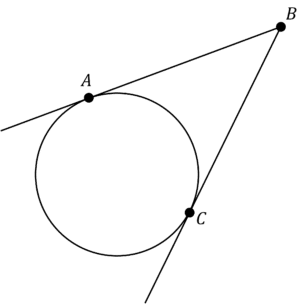[1 mark]

This is a simple question as we can quickly apply only one rule: rule 3.

As these tangents come from the same point, there is the same length between each of the lines meeting and the circumference. Therefore, length $BC$ is also $7.5\text{ cm}$

Level 4-5GCSEEdexcel iGCSE

## Example 2: Using Multiple Circle Theorems

You may also come across problems where you need to use multiple circle theorems to find the solutions.

Find the size of angle $AOC$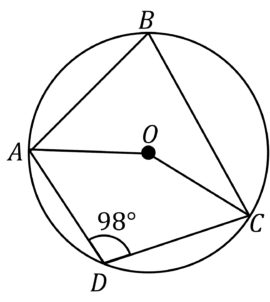[4 marks]

Immediately, we cannot find the angle at the centre. Let’s consider which rules would allow us to find the angle at the centre…

Rule 6 allows us to find the angle at the centre, but to do this, we will need the angle subtended on the circumference, $ABC$

So how can we find this angle? The shape within the circle is a cyclic quadrilateral, and the angle we have been given, $ADC$ is opposite the angle we want to find, $ABC$. And, using rule 7, we know these angles add to $180\degree$

Therefore, angle $ABC$,

$180-98=82\degree$

Now we can go back to rule 6, and we know the angle at the centre is double the angle at the circumference, so angle $AOC$,

$2\times 82 = 164\degree$

Level 8-9GCSEEdexcel iGCSE

## Circle Theorems Example Questions

We can use rule 2 here. A tangent and a radius will always meet at $90\degree$. Therefore,

Angle $ACO$ is $90\degree$

Even though the chords are extended here, they still intersect within the circle, and so we use rule 9 part 1, internal intersection,

$AE\times EB = CE\times ED$

Make sure you check that the $A, B, C, D$ in your diagram match up to those in the rule, or change them accordingly. In this case, the diagram does match up, so:

$4\times EB = 12\times6\\$

$4\times EB=72$

$EB=18\text{ cm}$

You may notice this diagram is a triangle met with a tangent, similarly to the alternate segment theory (rule 8), and so, we’ll start by using this.

This rule tells us that angle $BCE$ is  $38\degree$

Now, we can employ another rule.

As the tangent is meeting a radius, we know angle $OCE$ is $90\degree$ (using rule 2). This means we can work out $x$,

$x=90-38=52\degree$

Firstly, we can notice that the line going through the chord is a perpendicular bisector to the chord – it has a right angle and splits the chord into two equal lengths. And so, using rule 1, this bisector must pass through the centre.

This means this line is also a diameter.

Thus, we can use rule 4, an angle subtended from the diameter is always $90\degree$. Therefore, $x=90\degree$

## You May Also Like...### MME Learning Portal

Online exams, practice questions and revision videos for every GCSE level 9-1 topic! No fees, no trial period, just totally free access to the UK’s best GCSE maths revision platform.

£0.00